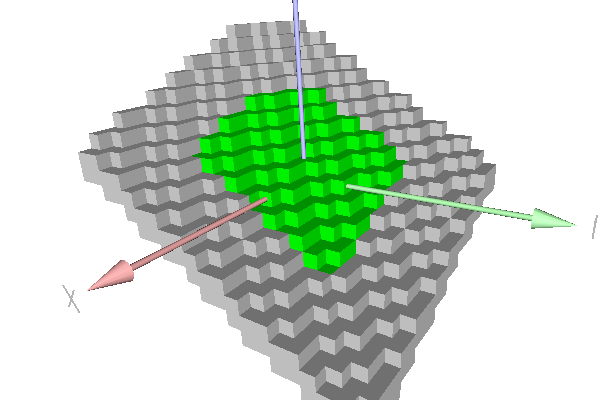DGtal  1.4.beta
io/viewers/viewer3D-7bis-stdplane.cpp

This example shows the recognition of a simple standard digital plane with the Chord algorithm (class ChordGenericStandardPlaneComputer ). Green points belong to the naive plane. Grey points show farther points that also belong to the strip.

Standard plane recognition with Chord algorithm
\$ ./examples/io/viewers/viewer3D-7bis-stdplanePiece of standard plane.
#include <cstdlib>
#include <iostream>
#include "DGtal/base/Common.h"
#include "DGtal/helpers/StdDefs.h"
#include "DGtal/geometry/surfaces/ChordGenericStandardPlaneComputer.h"
#include "DGtal/io/viewers/Viewer3D.h"
using namespace std;
using namespace DGtal;
// Standard services - public :
/*
Displays in the \a viewer all the points in the \a domain that
satisfies the predicate \a pred.
*/
template <typename Viewer3D, typename Domain, typename Predicate>
void
displayPredicate( Viewer3D & viewer,
const Domain & domain, const Predicate & pred )
{
for ( typename Domain::ConstIterator itB = domain.begin(), itE = domain.end();
itB != itE; ++itB )
{
if ( pred( *itB ) )
viewer << *itB;
}
}
/*
Displays in the \a viewer all the points in the range [it,itE).
*/
template <typename Viewer3D, typename InputIterator>
void
displayRange( Viewer3D & viewer, InputIterator it, InputIterator itE )
{
for ( ; it != itE; ++it )
viewer << *it;
}
/*
Returns all the points in the \a domain that belongs to the standard
plane mu <= a*x+b*y+c*z < mu+|a|+|b|+|c|.
*/
template <typename Domain>
std::vector<typename Domain::Point> pointsInStandardPlane
( const Domain & domain,
typename Domain::Integer a,
typename Domain::Integer b,
typename Domain::Integer c,
typename Domain::Integer mu )
{
typedef typename Domain::Integer Integer;
typedef typename Domain::Point Point;
std::vector<Point> pts;
Integer mup = mu + abs(a) + abs(b) + abs(c);
for ( ConstIterator it = domain.begin(), itE = domain.end();
it != itE; ++it )
{
Point p = *it;
Integer r = a * p[ 0 ] + b * p[ 1 ] + c * p[ 2 ];
if ( ( mu <= r ) && ( r < mup ) )
pts.push_back( p );
}
return pts;
}
int main( int argc, char** argv )
{
using namespace Z3i;
unsigned int nb = 0;
unsigned int nbok = 0;
QApplication application(argc,argv);
unsigned int diameter = argc > 1 ? atoi( argv[ 1 ] ) : 10;
int a = argc > 2 ? atoi( argv[ 2 ] ) : 2;
int b = argc > 3 ? atoi( argv[ 3 ] ) : 3;
int c = argc > 4 ? atoi( argv[ 4 ] ) : 5;
int mu = argc > 5 ? atoi( argv[ 5 ] ) : 0;
trace.beginBlock ( "Testing class ChordGenericStandardPlaneComputer" );
trace.info() << "Recognizing plane "
<< mu << " <= " << a << " * x + "
<< b << " * y + " << c << " * z < "
<< (mu+abs(a)+abs(b)+abs(c)) << std::endl;
Domain domain1( Point( -diameter, -diameter, -diameter ),
Point( diameter, diameter, diameter ) );
typedef int64_t Integer;
typedef ChordGenericStandardPlaneComputer<Z3, Point, Integer> PlaneComputer;
typedef PlaneComputer::Primitive Primitive;
PlaneComputer plane;
plane.init( 1, 1 );
std::vector<Point> recognized = pointsInStandardPlane( domain1,
a, b , c, mu );
++nb, nbok += plane.extend( recognized.begin(), recognized.end() ) ? 1 : 0;
trace.info() << "(" << nbok << "/" << nb
<< ") All points are recognized." << std::endl;
trace.info() << " - Plane=" << plane
<< std::endl;
Primitive strip = plane.primitive();
trace.info() << "strip=" << strip
<< " axis=" << strip.mainAxis()
<< " axiswidth=" << strip.axisWidth()
<< " diag=" << strip.mainDiagonal()
<< " diagwidth=" << strip.diagonalWidth()
<< std::endl;
++nb, nbok += ( strip.diagonalWidth() < sqrt(3.0) ) ? 1 : 0;
trace.info() << "(" << nbok << "/" << nb
<< ") Diagonal width < sqrt(3)." << std::endl;
trace.emphase() << ( (nb == nbok) ? "Passed." : "Error." ) << endl;
typedef Viewer3D<> MyViewer;
MyViewer viewer;
viewer.show();
Color red( 255, 0, 0 );
Color green( 0, 255, 0 );
Color grey( 200, 200, 200 );
Domain domain2( Point( -2*diameter, -2*diameter, -2*diameter ),
Point( 2*diameter, 2*diameter, 2*diameter ) );
viewer << CustomColors3D( red, red );
for ( std::vector<Point>::const_iterator it = recognized.begin(),
itE = recognized.end(); it != itE; ++it )
if ( ! strip( *it ) ) viewer << *it;
viewer << CustomColors3D( green, green );
displayRange( viewer, plane.begin(), plane.end() );
viewer << CustomColors3D( grey, grey );
displayPredicate( viewer, domain2, strip );
viewer << MyViewer::updateDisplay;
trace.info() << "- Points in green have been recognized as belonging to this standard plane." << std::endl;
trace.info() << "- Points in grey belongs also to the parallel strip of the recognized standard plane." << std::endl;
trace.info() << "- Points in red belongs to the parallel strip of the recognized standard plane but not to the input standard plane: NONE should be red." << std::endl;
return application.exec();
}
// //
void beginBlock(const std::string &keyword="")
std::ostream & emphase()
std::ostream & info()
double endBlock()
Point::Coordinate Integer
MyDigitalSurface::ConstIterator ConstIterator
DGtal is the top-level namespace which contains all DGtal functions and types.
boost::int64_t int64_t
signed 94-bit integer.
Definition: BasicTypes.h:74
Trace trace
Definition: Common.h:153
int main(int argc, char **argv)
std::vector< typename Domain::Point > pointsInStandardPlane(const Domain &domain, typename Domain::Integer a, typename Domain::Integer b, typename Domain::Integer c, typename Domain::Integer mu)
MyPointD Point
Definition: testClone2.cpp:383
void displayRange(const R &r)
Domain domain
HyperRectDomain< Space > Domain
void displayPredicate(Viewer3D &viewer, const Domain &domain, const Predicate &pred)